# Lua中的常用操作系统库

## os.time ([table])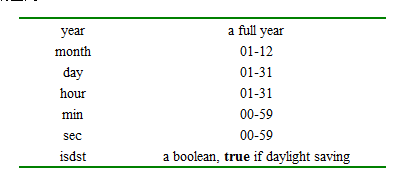``````print(os.time{year=1970, month=1, day=1,hour=8})

print(os.time{year=1970, month=1, day=1}) --若未定义“时，分，秒”,默认时间为正午（04:00:00）``````

-->0

-->14400(14400 = 46060 )

## os.date ([format [, time]])

*t":将返一个带year(4位),month(1-12), day (1--31), hour (0-23), min (0-59), sec (0-61), wday (星期几, 星期天为1), yday (年内天数), and isdst (是否为日光节约时间true/false)的带键名的表;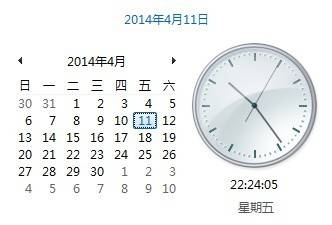``````t = os.date("*t", os.time());
for i, v in pairs(t) do
print(i,"->",v);
end``````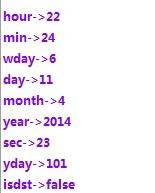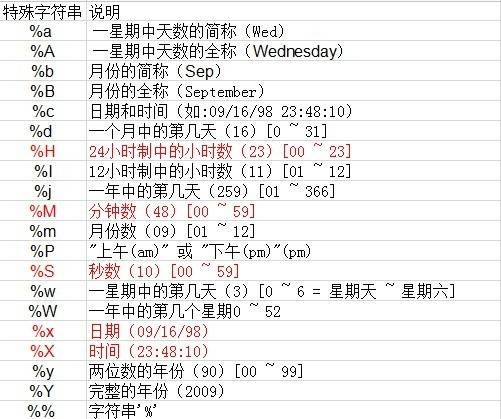``````print(os.date("today is %A, in %B"))

print(os.date("%X", 906000490))``````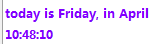``print(os.date("%m/%d/%Y", 906000490))``

<img src="/attachments/image/cimg/ph2os.difftime (t2, t1)/h2p功能：返回t1到t2相差的秒数 /pp例子：/pprecodet1 = os.time();for i = 0, 100000 do os.time();endt2 = os.time();print(string.format(" t1:="" %d="" t2:="" %d",t1,t2))="" print(os.date("%x",="" t1))="" t2))="" print(os.difftime(t2,="" t1));="" code="" pre="" p运行结果：="" p="" pimg="" elapsed="" time="" :="" %.2f\n",="" os.clock()="" -="" x));<="">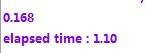## 您可能还喜欢：